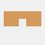# Interesting fact about perfect squares

Hi, I found out something interesting about perfect squares and I would like to share it.

I don't know if anyone knew this, but I believe that most of you might knew this.

This is a quite easier way to find the square of a large number

Alright, let's start with two unknowns. I'll take $x$ and $y$ while $y = x+1$

As we knew that $x^2 = x\times x$ and $y^2 = y\times y$, we can change $y^2$ to be

$y\times y = y(x+1) = y\times x + y$

$=x(x+1) + y = x\times x + x + y$

$=x^2 + x + y$

$y^2 = x^2+x+y$

Now, we have a simplified version of $y^2$

Let's try $x = 3$ and $y = 4$

$4^2 = 3^2 + 3 + 4$

$16 = 9 + 3 + 4$

And 16 is indeed equal to $9 + 3 + 4$

So it worked! Yeah!

Now let's try a larger number, 501

If $y = 501, x = 500$

$501^2 = 500^2 + 500 + 501$

$501^2 = 250000 + 500 + 501$

$501^2 = 251001$

Try it in your calculator and you will find it true

Alright, that's all. Thanks everyone for viewing this.

Try this problem to see if you understand it

Follow me to get more interesting questions and notesNote by Daniel Lim
7 years, 4 months ago

This discussion board is a place to discuss our Daily Challenges and the math and science related to those challenges. Explanations are more than just a solution — they should explain the steps and thinking strategies that you used to obtain the solution. Comments should further the discussion of math and science.

When posting on Brilliant:

• Use the emojis to react to an explanation, whether you're congratulating a job well done , or just really confused .
• Ask specific questions about the challenge or the steps in somebody's explanation. Well-posed questions can add a lot to the discussion, but posting "I don't understand!" doesn't help anyone.
• Try to contribute something new to the discussion, whether it is an extension, generalization or other idea related to the challenge.

MarkdownAppears as
*italics* or _italics_ italics
**bold** or __bold__ bold
- bulleted- list
• bulleted
• list
1. numbered2. list
1. numbered
2. list
Note: you must add a full line of space before and after lists for them to show up correctly
paragraph 1paragraph 2

paragraph 1

paragraph 2

[example link](https://brilliant.org)example link
> This is a quote
This is a quote
    # I indented these lines
# 4 spaces, and now they show
# up as a code block.

print "hello world"
# I indented these lines
# 4 spaces, and now they show
# up as a code block.

print "hello world"
MathAppears as
Remember to wrap math in $$ ... $$ or $ ... $ to ensure proper formatting.
2 \times 3 $2 \times 3$
2^{34} $2^{34}$
a_{i-1} $a_{i-1}$
\frac{2}{3} $\frac{2}{3}$
\sqrt{2} $\sqrt{2}$
\sum_{i=1}^3 $\sum_{i=1}^3$
\sin \theta $\sin \theta$
\boxed{123} $\boxed{123}$

Sort by:

Why complicate the matter when you have got such a nice formula that you have been learning since "AGES"..... (a^ {2} - b^ {2} ) = (a-b)(a+b)

- 7 years, 4 months ago

You can also think of it as this: Suppose x is 10 and y is 11. Imagine you have a square of 100 counters laid out in a 10 by 10 fashion. By adding 10 counters to the right of your grid, you get 110 counters in an 11x10 fashion. Then, add 11 counters to the bottom of the new grid and you get an 11 by 11 SQUARE grid. This shows that x^2+x+y=y^2. This also works for all consecutive numbers (you can try it yourself with counters.) Just another way to think of this method as a whole without using any algebra.

- 7 years, 4 months ago

That was the way I learnt it 0.o

- 6 years, 12 months ago

a^2- b^2 = (a+b)(a-b), an EASIER way!

- 6 years, 1 month ago

This works is because $(x-1)^2-x^2=2x-1$, and as we know, all odds can be written as the sum of two consecutive numbers. But this do save a little time. How about this?

For every two digit which has the form of $\overline {a5}$, when they multiply with another number with the same form, then, $\overline {a5} \times \overline {b5} = \overline{wxyz}$, $\overline {wx} = a(a+1), \overline {yz} = 25$.

- 7 years, 4 months ago

don't understand the $a5 b5$ part

- 7 years, 4 months ago

I want to clarify that $\overline{a5}$ is not $a \times 5$, but $\overline {a5} = 10a + 5$.

- 7 years, 4 months ago

Well it isn't a simplification since we have to do 3 more operations instead of just one.

- 6 years, 12 months ago

(a + 1)^2 = a^2 + 2a + 1 = a^2 + a + (a + 1) just what you had above. Let us see a two digit number.
(10a + b)^2 = 100a^2 + b^2 + 20ab........... (10a - b)^2 = 100a^2 + b^2 - 20ab
Say 63^2 = (60+3)^2 = 3609 + 2018 = 3969 ...............67^ 2 = (70 - 3)^2 = 4909 - 2073 = 4489.
If b < 5 use (10a + b)^2, b > 5 use {(10 +1)a - (10 - b) }^2
If b=5, (10a + 5)^2 = 100
a*(a+1) +25

- 6 years, 11 months ago